Duan, R., Wang, Y., Zhao, L., Yun, X. D., and Zhou, N. (2022). "Prediction of wood moisture content based on THz time-domain spectroscopy," BioResources 17(3), 4745-4762.

#### Abstract

A new method for predicting wood moisture content using terahertz (THz) time-domain spectroscopy (TDS) is presented in this paper. The THz wave is a promising method in measuring wood moisture content due to its sensitivity to water, impressive penetration ability in wood, and no destructive effect on wood interior. In this study, the selected wood, Douglas fir (Pseudotsuga menziesii), with different moisture content was studied. THz-TDS was used to extract the optical parameter of the sample. The THz refractive index and absorption coefficient spectrum of the wood were calculated. The first and second derivatives of the absorption coefficient spectrum were processed to obtain the first and second derivative spectra. The successive projections algorithm (SPA) was used to select the characteristic frequency for the THz absorption coefficient spectrum and its first and second derivative spectrum of the wood. A regression prediction model of wood moisture content was established by partial least squares regression (PLS). The results showed that the proposed model based on the second derivative spectrum had the best prediction effect for the moisture content of wood.

Prediction of Wood Moisture Content Based on THz Time-Domain Spectroscopy

Ruifeng Duan,a Yuan Wang,b,c,d,* Lei Zhao,b,c,d Xing Da Yun,b,c,d and Nan Zhou e

A new method for predicting wood moisture content using terahertz (THz) time-domain spectroscopy (TDS) is presented in this paper. The THz wave is a promising method in measuring wood moisture content due to its sensitivity to water, impressive penetration ability in wood, and no destructive effect on wood interior. In this study, the selected wood, Douglas fir (Pseudotsuga menziesii), with different moisture content was studied. THz-TDS was used to extract the optical parameter of the sample. The THz refractive index and absorption coefficient spectrum of the wood were calculated. The first and second derivatives of the absorption coefficient spectrum were processed to obtain the first and second derivative spectra. The successive projections algorithm (SPA) was used to select the characteristic frequency for the THz absorption coefficient spectrum and its first and second derivative spectrum of the wood. A regression prediction model of wood moisture content was established by partial least squares regression (PLS). The results showed that the proposed model based on the second derivative spectrum had the best prediction effect for the moisture content of wood.

DOI: 10.15376/biores.17.3.4745-4762

Keywords: Wood non-destructive testing; Water content prediction; Terahertz; Spectral analysis

Contact information: a: School of Information Science and Technology, Beijing Forestry University, Beijing 100083, P.R. China; b: School of Technology, Beijing Forestry University, Beijing 100083, P.R. China; c: Key Lab of State Forestry and Grassland Administration for Forestry Equipment and Automation, Beijing 100083, P.R. China; d: Joint International Research Institute of Wood Nondestructive Testing and Evaluation, Beijing Forestry University, Beijing 100083, P.R. China; e: State Key Laboratory of Precision Measurement Technology and Instrument, Tianjin University, Tianjin 300072, P.R. China;

* Corresponding author: wangyuan@bjfu.edu.cn

INTRODUCTION

Wood drying is an essential procedure to improve wood quality and utilization rate. In this process, wood moisture content is a key parameter because one can choose a scientific drying technology. For instance, when the moisture content of wood is high, it is necessary to use lower temperature and higher humidity. When the moisture content is approximately 30%, the drying must be carried out slowly. Drying without knowing the moisture content of the wood may lead to cracking, warping, and wood defects. Therefore, it is necessary to detect wood moisture content accurately ahead of time.

Traditional methods of wood moisture content detection include the weighing method, conductivity method, capacitance method, and microwave method (Schajer et al. 2006; Zhou et al. 2011; Koumbi-Mounanga et al. 2015). The weighing method can obtain accurate measurement results. However, the testing process is time-consuming and greatly influenced by human factors. The resistance method determines the wood moisture content by measuring the resistance between two electrodes, which is based on the conductivity of moisture in wood. When measuring the moisture content near the fiber saturation point, the measured value will suddenly deviate from the true value. The microwave method is used to measure the wood moisture content, and the relationship between the microwave and the wood moisture content can be determined. However, the measurement range is limited and affected by the temperature and humidity in the wood drying room, which leads to a large measurement error. Therefore, developing a non-contact and non-destructive detecting approach to obtain accurate wood moisture content during the drying process is an important issue that urgently needs to be solved.

Many researchers resort to spectral analysis methods due to their characteristics of rapidity and non-destructiveness. The nuclear magnetic resonance (NMR) (Almeida et al. 2007; Xu et al. 2017) method has high precision and speed, but it has a requirement on the size of the sample and is expensive. Near infrared (NIR) spectroscopy (Watanabe et al. 2011; Kobori et al. 2013) can also be utilized to measure wood water content rapidly and nondestructively, but the poor penetration makes it helpless for measuring the inside of the wood. X-rays (Tanaka et al. 2009; Kim et al. 2015) have outstanding penetration in wood, but they are harmful to the human body.

Terahertz (THz) spectroscopy has emerged as an important spectral analysis technique in recent years. The THz spectrum contains abundant physical and chemical information of the measured object. The weak intermolecular interactions, such as van der Waals force and hydrogen bond, as well as the molecular structure and related environmental information reflected by the skeleton vibration, dipole vibration, rotation transition of many macromolecules, and the low-frequency vibration of crystal lattice, have obvious response to the absorption intensity and the location of absorption peak in THz absorption spectrum (Zhang et al. 2007a). The THz time-domain spectroscopy (THz-TDS) spectrum can be used to calculate the optical parameters, such as the absorption coefficient, refractive index, and dielectric constant, of the sample. These parameters can be used to analyze the composition, structure, physical and chemical properties of the materials. Due to its strong correlation to water and good penetration in wood, THz wave is suitable for wood moisture content detection.

In this paper, THz-TDS technique was used to predict the water content of Douglas fir. The authors collected THz time-domain spectra at different moisture content of the wood and calculated its absorption coefficient and refractive index spectra. It was found that the absorption coefficient spectrum had a strong relationship with water content. Furthermore, the first and second derivatives of the absorption coefficient spectrum were processed. Then, the successive projections algorithm (SPA) was used to select the characteristic frequency and the partial least squares (PLS) was used to establish a regression prediction model. The results showed that the SPA-PLS model can accurately detect the moisture content of wood and the model based on the second derivative spectrum displayed the best prediction effect.

ALGORITHM THEORY

Successive Projections Algorithm

The SPA is a forward variable selection algorithm to minimize the collinearity of vector space, which was proposed by Bregman in 1965 (Almeida et al. 2018). The advantage of this method is that it can extract several characteristic wavelengths of the whole band, thus eliminating redundant information in the original spectral matrix. The SPA is a cyclic variable selection method. The projection vectors of the selected frequencies on the unselected frequencies are computed cyclically, and the maximum frequency of the projection module is taken as the selected variable. Therefore, the correlation between the selected frequencies and the previous frequencies is the weakest. The SPA calculation is simple and fast. The method is generally used to select a characteristic wavelength of near infrared spectroscopy (ISO 3130 (1975)). In this study, the characteristic frequency of THz absorption coefficient spectrum and refractive index spectrum were screened by SPA.

Taking the spectral data set Xixj of j frequencies of i samples as an example, the steps of SPA are as follows:

(1) Before iteration, the m column of spectral training set data is assigned to xm(1), m ∊ 1, 2, … j.

(2) Set the unselected frequencies as S :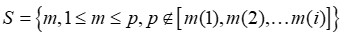(1)

(3) Calculate the projection of the selected frequency in the residual vector xk, and select the maximum frequency of the projection vector. Cyclic calculation until all frequencies have been calculated: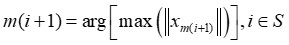(2)

(4) The multiple linear regression model is established by using the variables in the subset, and the subset with the minimum root mean square error is selected to carry out stepwise regression modeling, and the least characteristic variables are selected on the premise of guaranteeing accuracy.

Partial Least Squares

The PLS algorithm is a regression modeling method of multiple dependent variables to multiple independent variables, which realizes the combination of multiple linear regression, principal component analysis, and canonical correlation analysis.

The principle of PLS regression modeling is as follows: The sets of dependent variables and independent variables are Y = (y1, y2,…yq), yjRn, and X = (x1, x2,…xp), xiRn. Then, PLS extracts component t1 from X and component u1 from Y, where t1 is the linear combination of x1,x2,…xp, u1 is the linear combination of y1, y2,…yq. The extracted components should satisfy the following two requirements: Firstly, t1 and u1 should carry the variation information in their respective data tables to the greatest extent; Secondly, t1 and u1 have the greatest correlation. After the first components t1and u1 are obtained, the regressions of X to t1 and Y to u1 are calculated. If the accuracy of the regression equation does not meet the requirements, the residual information is used to extract the components. The m components t1, t2,…tm extracted by X are analyzed by yk(k = 1, 2…, q)regression method, and finally reduced to the equation of yk about x1 ,x2,…xp.

The PLS regression model needs principal component analysis. The determination of principal component has a great impact on the accuracy of the model. Predictive residual sum of squares (Sspre) and cross validation are usually used to find the optimal number of principal components. The smaller the Sspre value, the higher the prediction accuracy of the model is. The Sspre can be calculated by: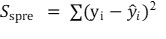(3)

where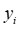is the standard data and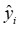is the predicted data.

Model Evaluation Index

The accuracy of the regression prediction model needs to be judged after it is established. The accuracy of the model is mainly evaluated through fitting degree, correlation coefficient, and root mean square error (RMSE) in this paper.

Fitting degree is expressed by R2, whose range is [0, 1]. The closer its value is to 1, the better the fitting degree of the model is. Fitting degree is given as follows,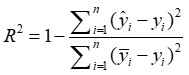(4)

where n is the number of sample, yi is the standard value, is the predicted value, and is the average of the standard value.

Variable stands for the correlation coefficient. If > 0, it indicates that the two variables are positively correlated. In contrast, if < 0 it means that they are negatively correlated. The closer approaches 1, the closer the linear relationship between the two variables is. The closer approaches 0, the weaker the linear correlation between the two variables is. Correlation coefficient is defined as Eq. 5,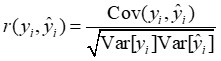(5)

where Cov(yi, ) is the covariance between yi and, and Var[yi] and Var[] are the variances of yi and , respectively.

The RMSE is used to measure the deviation between the observed value and the true value. The smaller the RMSE of the samples in the prediction set, the better the fitting and prediction effect of the model is. The RMSE is expressed as follows: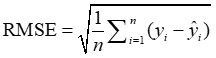(6)

EXPERIMENTAL

Douglas fir was selected to be the experimental material. The authors made 5 pieces of wood samples. The samples were cut transversely and the sizes of each sample were 50 mm × 30 mm × 5 mm.

Standard Moisture Content Determination Method

According to the method for determination of the density of wood (ISO 3131 (1975)), the wood samples were placed in a drying box and dried at 103 ± 2 ℃ for 8 h (Zhao et al. 2018). Then, an electronic balance with accuracy of 0.001 g was used to weigh the quality. During the drying course, wood samples were weighed every 2 h and THz time-domain spectrum was acquired after each weighing. The test ended when the change of sample weight was less than the sample mass, which means the wood was absolutely dried. The process is shown in Fig. 1.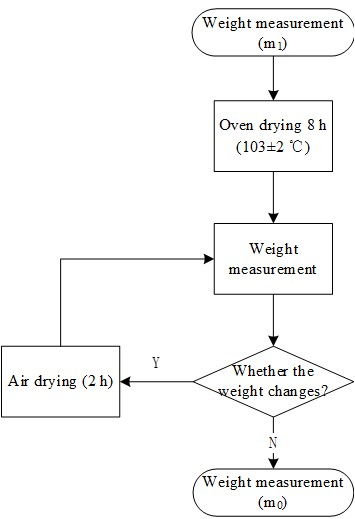Fig. 1. Wood moisture content measurement process

The moisture content of wood was calculated according to the following Eq. 7,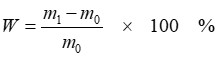(7)

where m1 is the sample weight (g) in the experiment and m0 is the sample weight (g) when absolutely dried.

THz Time-Domain Spectrum Acquisition

The THz-TDS is a coherent measurement technology of wideband THz pulse, which can detect the amplitude and phase information of THz pulse at the same time, and the spectral information can be achieved by Fourier transform infrared spectroscopy (Gribenyukov et al. 2018). It is convenient to extract the refractive index, absorption coefficient, extinction coefficient, and other optical parameters of the sample from the THz-TDS spectrum. Then, some physical properties and chemical information of samples can be obtained by analyzing these optical parameters.

According to the collection mode of THz spectrum, the THz-TDS system can be divided into transmission type and reflection type. In practical operation, the appropriate THz spectrum measurement method should be varied according to different samples and experimental conditions. When the tested samples are thin or the absorption intensity to THz pulses is low, the THz time-domain spectra of samples can be better obtained by transmission type. The THz time-domain spectroscopy system used in this paper is the transmission type.

In this paper, the THz spectra of wood samples were collected by the THz-TDS equipment (MenloSystems, Martinsried, Germany) in the State Key Laboratory of Precision Measurement Technology and Instruments of Tianjin University. The operating wavelength range of the equipment is 780 to 1650 nm, pulse width is 90 fs, total average output power is 500 mw, and repetition rate is 100 mHz. The block diagram of the THz-TDS system is shown in Fig. 2.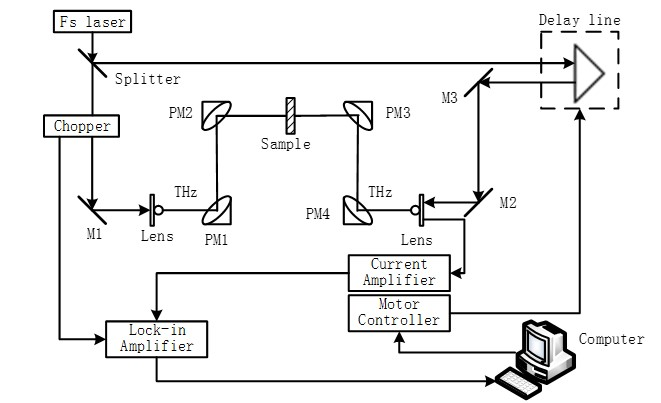Fig. 2. THz-TDS system diagram

The wood samples were placed at the communal focus of parabolic mirror PM2 and PM3 of THz-TDS system, and the THz wave was perpendicular to the wood texture. The transmission spectra of samples can be obtained by a scanning operation. A set of reference spectra with no samples were measured before the scanning operation. The frequency-domain spectra of the samples and reference signals were obtained after fast Fourier transform (FFT) was applied to the time-domain signal (TERA K15; MenloSystems, Martinsried, Germany).

RESULTS AND DISCUSSION

THz Spectral Analysis

The original THz time-domain spectra of wood were obtained by transmission of THz-TDS. In order to reduce the influence of ambient noise, each sample was measured three times and the average value was taken as the detection result. A total of 200 groups of wood THz spectra below fiber saturation point were obtained in the test, including moisture content ranged from 1.29% to 35.49%.

Five kinds of wood THz spectra with different moisture contents were selected to display how the spectral waveform change with moisture content, which is shown in Fig. 3. Compared with the reference spectra, the THz time-domain spectra of wood samples had a certain delay in time and an attenuation in amplitude. The time delay increased along with the moisture content of wood samples, while the amplitude decreased with it. In order to eliminate the measurement errors caused by factors such as equipment accuracy, water vapor in air, and the oscillation caused by reflection and refraction of THz wave, the wood THz time-domain spectral data were intercepted by Windowing.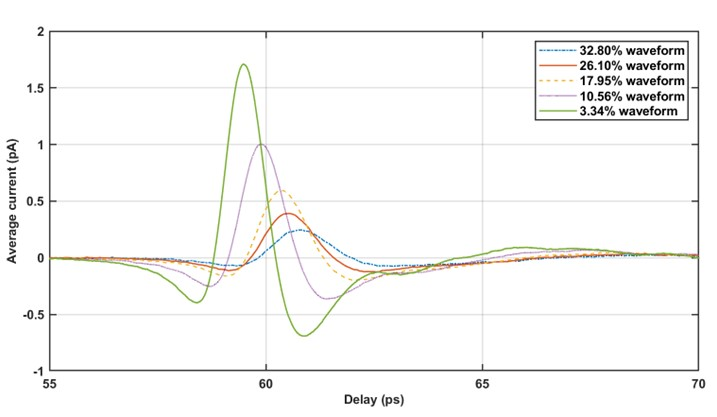Fig. 3. THz time-domain waveform of wood samples with different moisture content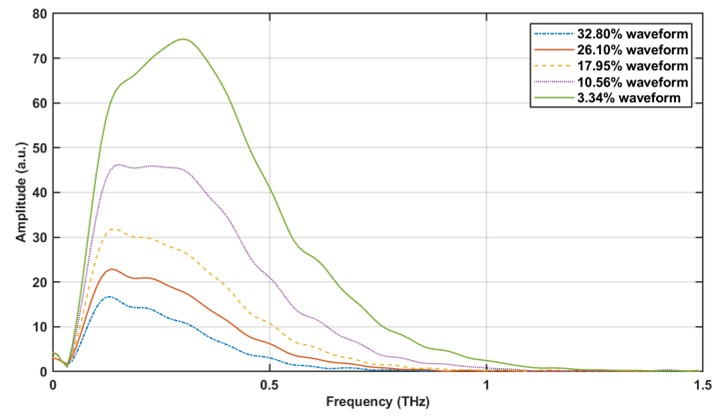Fig. 4. THz frequency-domain waveform of wood samples with different moisture content

The THz frequency-domain spectra of wood samples can be obtained by performing FFT to the time-domain spectra, which is shown in Fig. 4. It can be seen that the trend of the spectral waveform of wood samples with different moisture content was similar. However, the amplitudes were quite different. With the increase of moisture content of wood samples, the THz spectrum amplitude of wood samples decreased.

In conclusion, the THz time-domain waveform of wood samples with different moisture content showed different time delay and amplitude attenuation. The amplitude was negatively correlated with the moisture content of wood samples, while the time delay was positively correlated with it. In frequency-domain, the THz waveform of wood samples further showed the amplitude-frequency characteristics with different moisture content. The amplitude was negatively correlated with the moisture content of wood samples. It showed that it was feasible to use THz wave to predict wood moisture content.

THz Optical Parameter Extraction

According to the optical constants extracting model based on THz time-domain spectroscopy proposed by Dorney and Duvillaret, the absorption coefficient and refractive index can be calculated based on THz frequency (Li et al. 2014).

Macroscopic optical properties of samples can be described by complex refractive index,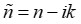(8)

where ñ is complex refractive index and n is real refractive index, which is used to describe the dispersion characteristics of samples, and k is extinction coefficient, which is used to describe the absorption characteristics of samples.

The refractive index of THz spectrum can be expressed as follows,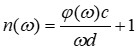(9)

and the expression of the absorbance is shown below in Eq. 10,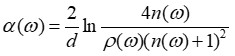(10)

where n(ω) is the real part of refractive index, d denotes the sample thickness (m), c is the velocity (m/s) of the THz wave propagating in a vacuum, ω is angular frequency, and ρ(ω) and φ(ω) represent the amplitude ratio and phase difference between sample signal and reference signal, respectively.

The absorption coefficient and refractive index spectrum are shown in Fig. 5 and Fig. 6. It can be seen from Fig. 5 that THz absorption coefficient spectrum was greatly influenced by water content. Though the waveform trends and the position of absorption peaks were basically the same, there were quite differences in the absorption intensity. The THz absorption intensity of wood enhanced with the increase of moisture content, indicating that the THz absorption intensity of wood was positively correlated with the moisture content of wood. In Fig. 6, the refractive index spectra of different water content seemed similar in appearance, no clear pattern was found. Therefore, the authors chose the THz absorption coefficient spectrum to predict the moisture content of wood in this paper.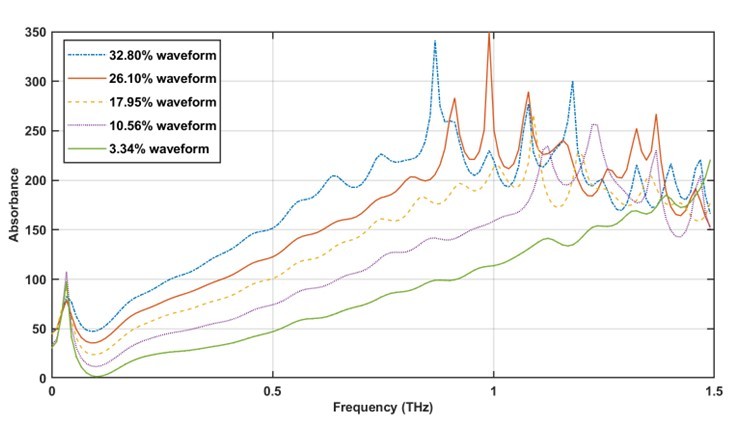Fig. 5. Waveform of THz absorption coefficient of wood samples at different moisture contents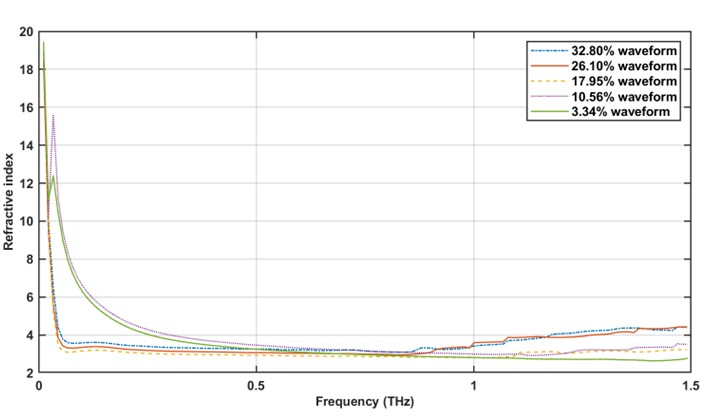Fig. 6. THz refractive index waveform of wood samples with different moisture content

Spectral Derivative Processing

To eliminate the baseline drift of the spectrum, enhance the spectral characteristics, and reduce the noise influence of the instrument itself, the first derivative and the second derivative of the absorption coefficient spectrum were processed to obtain the first derivative spectrum and the second derivative spectrum, as shown in Figs. 7 and 8.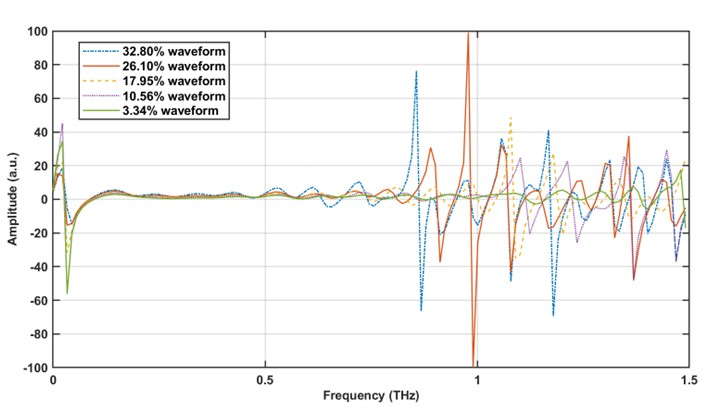Fig. 7. First derivative waveform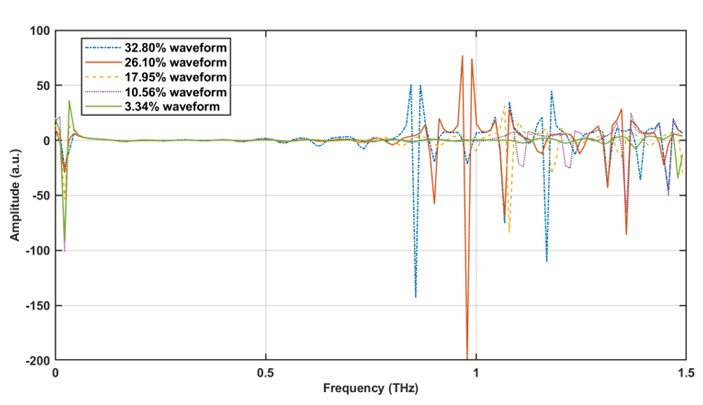Fig. 8. Second derivative waveform

Spectral Characteristic Selection

The characteristic frequencies of THz absorption coefficient spectrum, first derivative spectrum, and second derivative spectrum were selected by SPA. As shown in Fig. 9, seven characteristic frequency points were selected from the135 frequency points of absorption coefficient spectrum, accounting for 5.18% of all frequency bands. Figure 10 shows the variation of RMSE value with the increase of the number of variables. It can be seen that the RMSE decreased with the increase of variables at the beginning. When the number was 7, the RMSE reduced to 0.02421 and tended to be stable.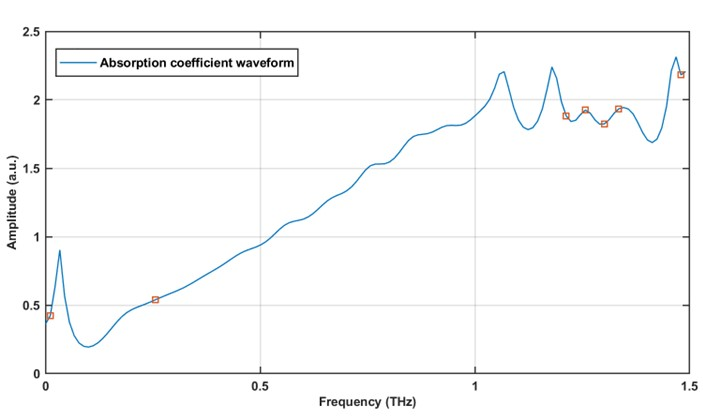Fig. 9. Characteristic frequencies selection of absorption coefficient spectrum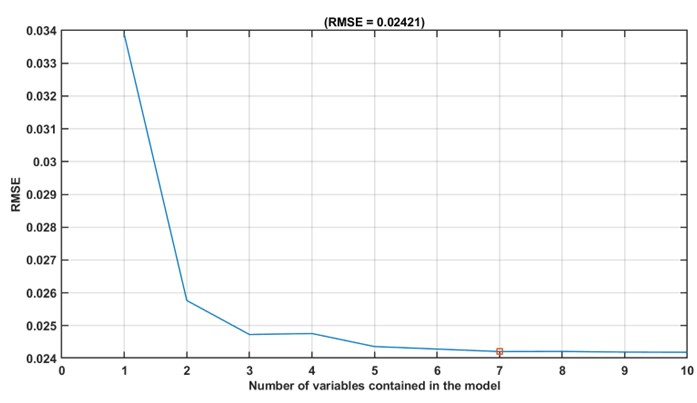Fig. 10. Variation of RMSE with the number of variables in absorption coefficient spectrum

The SPA screened nine characteristic frequency points from a total 135 frequency points of first derivative spectrum, accounting for 6.67% of all frequency bands, which is shown in Fig. 11. The RMSE was 0.0362, and the RMSE variation with the number of characteristic variables is shown in Fig. 12.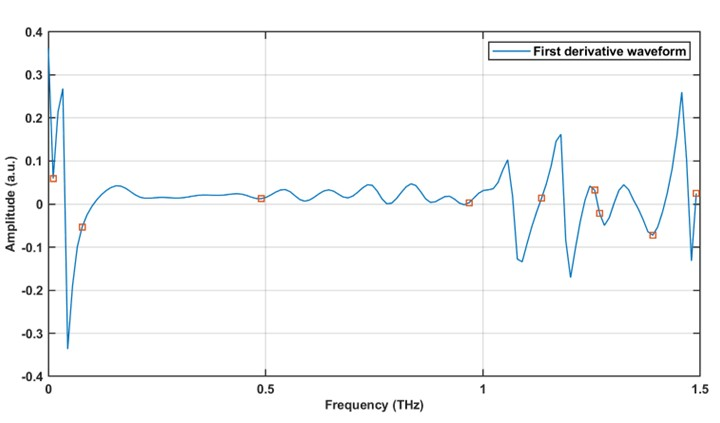Fig. 11. Characteristic frequency selection of first derivative spectrum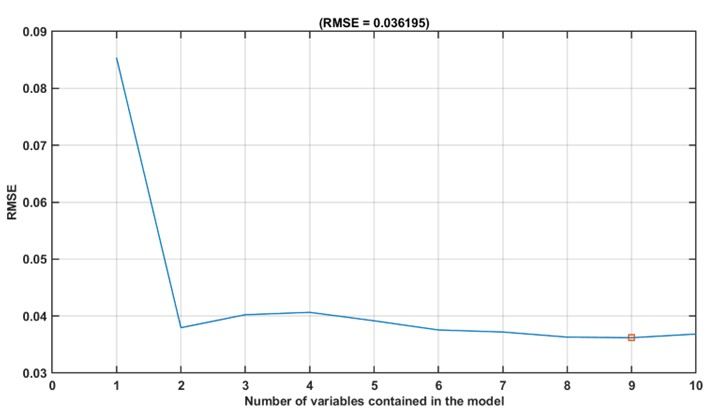Fig. 12. Variation of RMSE with the number of variables in first derivative spectrum

As shown in Fig. 13, approximately 16 characteristic frequency points were screened from 135 frequency points of second derivative spectrum, accounting for 11.85% of all frequency bands. The RMSE was 0.0364, and the RMSE variation with the number of characteristic variables is shown in Fig. 14.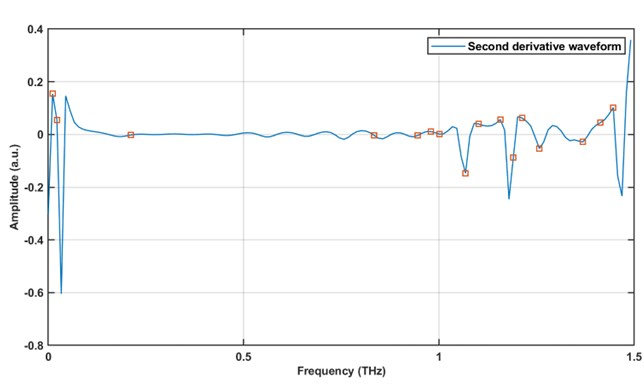Fig. 13. Characteristic frequency selection of second derivative spectrum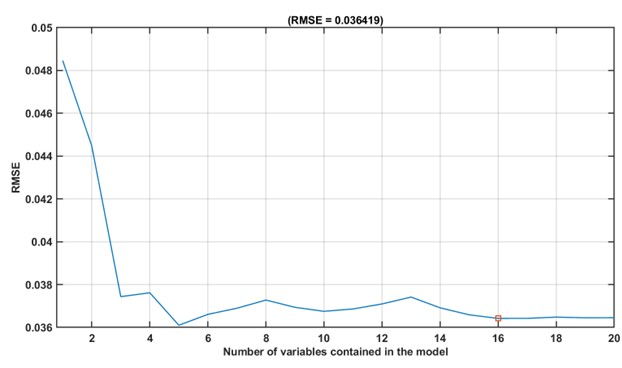Fig. 14. Variation of RMSE with the number of variables in second derivative spectrum

PLS Modeling and Evaluation

The authors respectively divided 200 sets of THz absorption coefficient spectra, first derivative spectra, and second derivative spectra into two groups, 150 groups as training set and 50 groups as test set. The PLS regression prediction model was established using training set, then the data of test set are predicted.

The PLS prediction model of wood moisture content was established based on THz absorption coefficient spectrum, first derivative spectrum, and second derivative spectrum. Figures 15, 16, and 17 show the scatter plots of PLS model based on training set and test set of three kinds of spectra, respectively.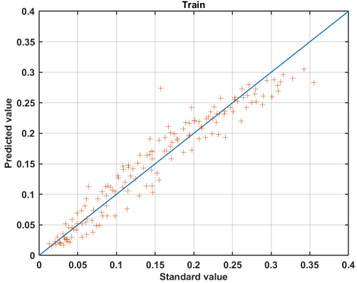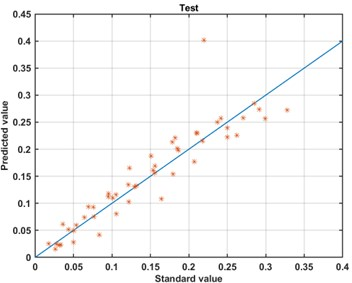Fig. 15. Scattered plot of absorption coefficient SPA-PLS prediction results based on training set and test set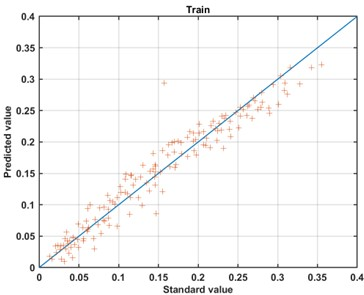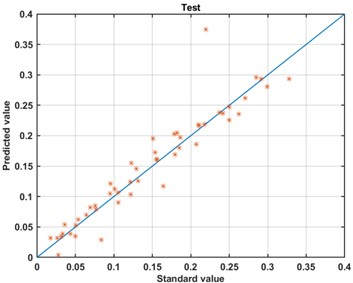Fig. 16. Scattered plot of first derivative SPA-PLS prediction results based on training set and test set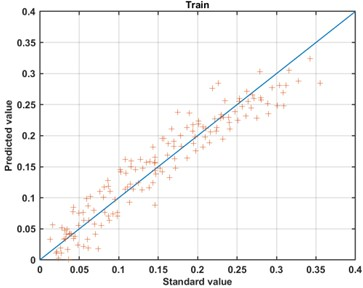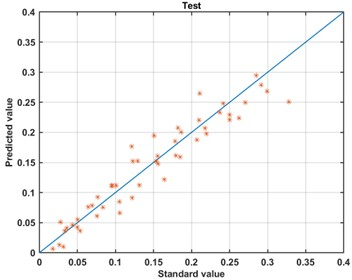Fig. 17. Scattered plot of second derivative SPA-PLS prediction results based on training set and test set

By analyzing the fitting results of scatter plots, the SPA-PLS prediction model based on the second derivative spectrum had the best fitting effect on the training set, while the absorption coefficient spectrum had the worst performance. The results showed that the derivative processing had an obvious effect on reducing noise and enhancing spectral characteristics, thus the prediction accuracy of the model was improved.

The fitting degree, correlation coefficient, and RMSE were used to evaluate the model, which is shown in Tables 1, 2, and 3. The results of training set and test set of PLS model were compared in different frequency bands of 0.2 to 1 THz and the feature frequencies screened by SPA.

Table 1. PLS Prediction Model Based on Absorption Coefficient